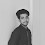# URI - BEECROWD - BEE Online Judge Solution  1005 | Average 1 - URI - BEECROWD - BEE Problem 1005 Solution in C,C++,Python

Read two floating points' values of double precision A and B, corresponding to two student's grades. After this, calculate the student's average, considering that grade A has weight 3.5 and B has weight 7.5. Each grade can be from zero to ten, always with one digit after the decimal point. Don’t forget to print the end of line after the result, otherwise you will receive “Presentation Error”. Don’t forget the space before and after the equal sign.

## Input

The input file contains 2 floating points' values with one digit after the decimal point.

## Output

Print the message "MEDIA"(average in Portuguese) and the student's average according to the following example, with 5 digits after the decimal point and with a blank space before and after the equal signal.

 Input Samples Output Samples 5.07.1 MEDIA = 6.43182
 0.07.1 MEDIA = 4.84091
 10.010.0 MEDIA = 10.00000

### URI Online Judge Solution  1005 | Average 1 - URI Problem 1005 Solution in C,C++,Python:

URI Problem 1005 Solution in C :

### 8 Responses to URI - BEECROWD - BEE 1005 | Average 1 - Solution in C,C++,Python

1.have a method to do this using threads?

2.Yes,you can try it.

3.why is it divided by 11??

4.It is divided by 11 because total weight is = 3.5+7.5=11 ... Hope you understand.

5.Can someone teach me the explanation behind this code ? why I have to multiply those weight with x & y?

6.You have to multiply those weight with x & y because if you are calculating GPA of student,then credit(here Weight) per subject is not same.

7.Why x & y, why not A & B?

8.same conditions for A & B . these are just variable names i used in C++ language.